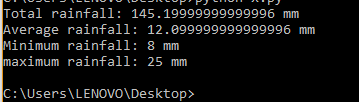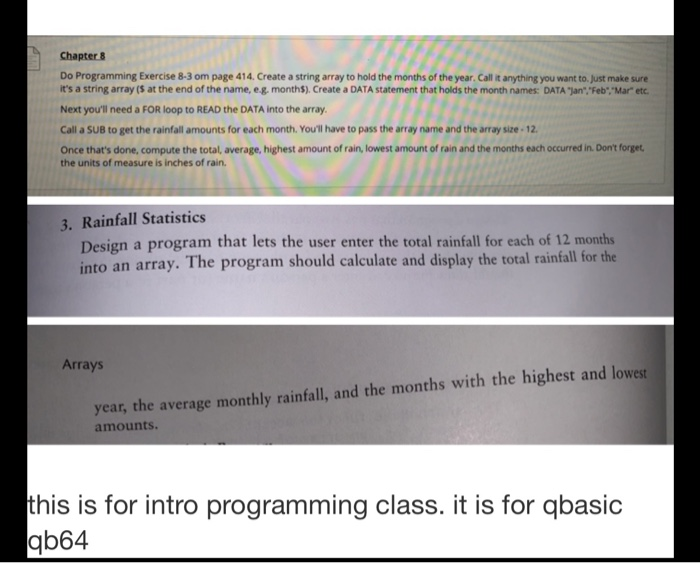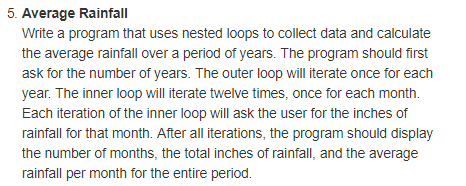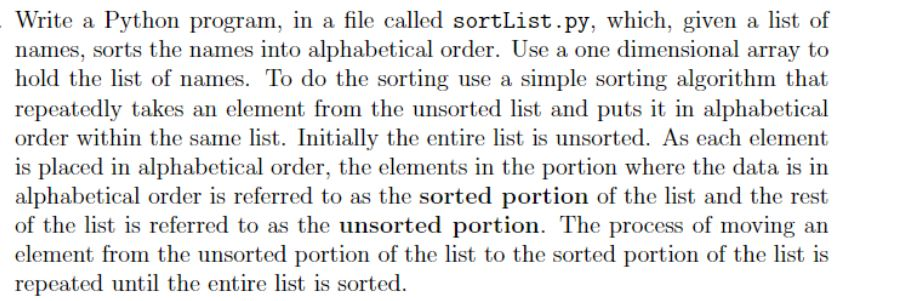Question

# Write a python program where you create a list (you an create it in code you...

Write a python program where you create a list (you an create it in code you do not have to use input() statements) that represents the total rainfall for each of 12 months (assume that element 0 is January and element 11 is December). The program should calculate and display the: 1) total rainfall for the year; 2) the average monthly rainfall; 3) the months with the highest and lowest amounts.

You need to figure out how to access each element in the list, You need to add the all up to find the total; you need to also divide by the length of your list to get the average; then use the min() and the max() functions to find the highest and lowest amounts.

Print out appropriate statements showing the 4 required outputs.

Code:

rainfall=[12.1,13.6,9.2,25,16,15.8,21,20,18.9,15,8,11] #list
totrainfall=0
for x in range(0,len(rainfall)): #iterating through every element of list
totrainfall=totrainfall+rainfall #adding up elements of list
avgrainfall=totrainfall/12
#printing information
print("Total rainfall:",totrainfall,"mm")
print("Average rainfall:",avgrainfall,"mm")
print("Minimum rainfall:",min(rainfall),"mm")
print("maximum rainfall:",max(rainfall),"mm")

Output:#### Earn Coins

Coins can be redeemed for fabulous gifts.

Similar Homework Help Questions
• ### Write a Python program that allows the user to enter the total rainfall for each of...

Write a Python program that allows the user to enter the total rainfall for each of 12 months into a list. The program should calculate and display the total rainfall for the year, the average monthly rainfall, and the months with the highest and lowest rainfall amounts. Data: January: 8.9 inches February: 11.1 inches March: 13.4 inches April: 6.9 inches May: 8.7 inches June: 9.1 inches July: 15.9 inches August: 4.4 inches September: 3.1 inches October: 5.6 inches November: 7.8...

• ### pseudocode and Python Repl.it :Recall that Programming Exercise 3 in Chapter 8 asked you to design...

pseudocode and Python Repl.it :Recall that Programming Exercise 3 in Chapter 8 asked you to design a program that lets the user enter the total rainfall for each of 12 months into an array. The program should calculate and display the total rainfall for the year, the average monthly rainfall, and the months with the highest and lowest amounts. Enhance the program so it sorts the array in ascending order and displays the values it contains.

• ### Can you help me write a Python 3.7 code for this question? Write a program using...

Can you help me write a Python 3.7 code for this question? Write a program using functions and mainline logic which prompts the user to enter a number, then generates that number of random integers and stores them in a list. It should then display the following data to back to the user: The list of integers The lowest number in the list The highest number in the list The total sum of all the numbers in the list The...

• ### qbasic is the programming language Chapter 8 Do Programming Exercise 8-3 om page 414. Create a...qbasic is the programming language Chapter 8 Do Programming Exercise 8-3 om page 414. Create a string array to hold the months of the year. Call it anything you want to just make sure it's a string array is at the end of the name, e.g. months). Create a DATA statement that holds the month names: DATA "jan","Feb", "Mar" etc. Next you'll need a FOR loop to READ the DATA into the array, Call a SUB to get the rainfall...

• ### Rainfall Statistics Write a program that lets the user enter the total rainfall for each of...

Rainfall Statistics Write a program that lets the user enter the total rainfall for each of 12 months (starting with January) into an array of doubles. The program should calculate and display (in this order): the total rainfall for the year, the average monthly rainfall, and the months with the highest and lowest amounts. Months should be expressed as English names for months in the Gregorian calendar, i.e.: January, February, March, April, May, June, July, August, September, October, November, December....

• ### Python Code Write a program using functions and mainline logic which prompts the user to enter...

Python Code Write a program using functions and mainline logic which prompts the user to enter a number. The number must be at least 5 and at most 20. (In other words, between 5 and 20, inclusive.) The program then generates that number of random integers and stores them in a list. The random integers should range from 0 to 100. (You can use a wider range if you want, but the lower end of the range must be at...

• ### Write them in python IDLE ***** 5. Average Rainfall Write a program that uses nested loops...Write them in python IDLE ***** 5. Average Rainfall Write a program that uses nested loops to collect data and calculate the average rainfall over a period of years. The program should first ask for the number of years. The outer loop will iterate once for each year. The inner loop will iterate twelve times, once for each month. Each iteration of the inner loop will ask the user for the inches of rainfall for that month. After all iterations,...

• ### Topics: list, file input/output (Python) You will write a program that allows the user to read...

Topics: list, file input/output (Python) You will write a program that allows the user to read grade data from a text file, view computed statistical values based on the data, and to save the computed statistics to a text file. You will use a list to store the data read in, and for computing the statistics. You must use functions. The data: The user has the option to load a data file. The data consists of integer values representing student...

• ### Design a program that lets the user enter the total rainfall for each of 12 months...

Design a program that lets the user enter the total rainfall for each of 12 months into an array. The program should calculate and display the total rainfall for the year, the average monthly rainfall, and the months with the highest and lowest amount. PLEASE MODULARIZED THE CODE   PLEASE USE C PROGRAMMING AND ADD PSEUDOCODE

• ### Python program - Write a Python program, in a file called sortList.py, which, given a list...Python program - Write a Python program, in a file called sortList.py, which, given a list of names, sorts the names into alphabetical order. Use a one dimensional array to hold the list of names. To do the sorting use a simple sorting algorithm that repeatedly takes an element from the unsorted list and puts it in alphabetical order within the same list. Initially the entire list is unsorted. As each element is placed in alphabetical order, the elements in...